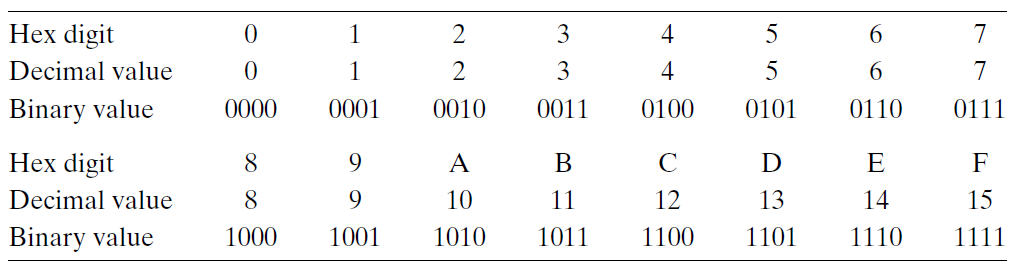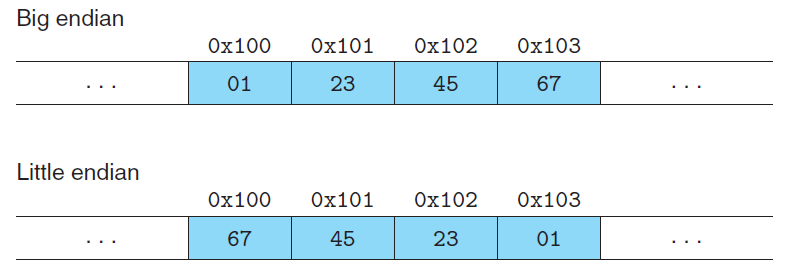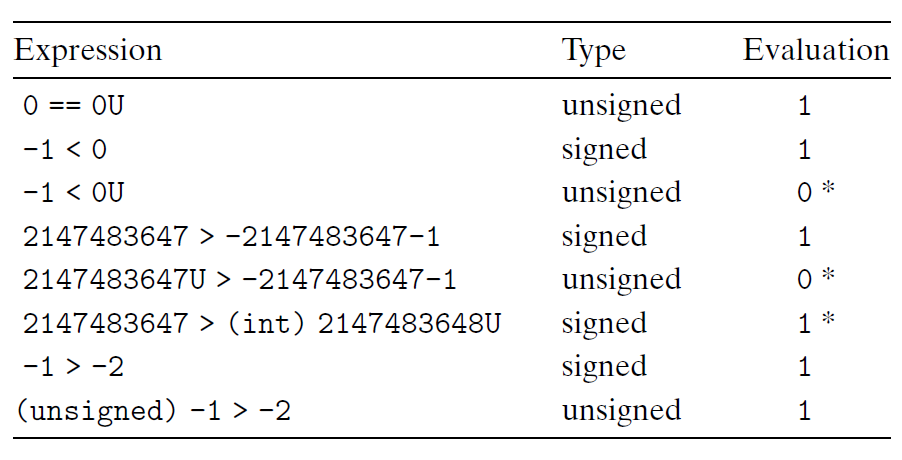# CSapp-Chapter2

Computer System A Programmers Perspective
This book is written from a programmer's perspective which describes how application programmers can use their knowledge of a system to write better programs.
Chapter 2

### Pre

• Unsigned encodings are based on traditional binary notation, representing numbers greater than or equal to 0.
• Two’s-complement encodings are the most common way to represent signed integers, that is, numbers that may be either positive or negative.
• Floating-point encodings are a base-two version of scientific notation for
representing real numbers.

### 2.1 Information Storage

Numeric constants starting with 0x or 0X are interpreted as being in hexadecimal, (or simply “hex”).#### Words

for a machine with a w-bit word size, the virtual addresses can range from 0 to 2w − 1, giving the program access to at most 2w bytes.

Little endian： The former convention—where the least significant byte comes first.(This convention is followed by most Intel-compatible machines.)
Big endian: The latter convention—where the most significant byte comes first.
Supposing the variable x of type int and at address 0x100 has a hexadecimal value of 0x01234567.(This convention is followed by most machines from IBM and Sun Microsystems.)Story:In fact, the terms “little endian” and “big endian” come from the book Gulliver’s Travels by Jonathan Swift, where two warring factions could not agree as to how a soft-boiled egg should be opened—by the little end or by the big.

#### Bit-Level Operations in C

As an application of the property that a ^ a = 0 for any bit vector a, consider the following program:

Steps x y
initialization 0100 0001 0101 1001
Steps 1 0100 0001 0001 1000
Steps 2 0101 1001 0001 1000
Steps 3 0101 1001 0100 0001

### 2.2 Integer Representations

#### Unsigned Encodings

$B2U_{w}$(for “binary to unsigned,” length w):

$B2U_{w}(\vec{x})\doteq\sum_{i=0}^{w-1}x_{i}2^i$

#### Two’s-Complement Encodings

$B2T_{w}$ (for “binary to two’s-complement” length w):

$B2T_{w}(\vec{x})\doteq-x_{w-1}2^{w-1}+\sum_{i=0}^{w-2}x_{i}2^i$

#### Signed vs. Unsigned in C

Although the C standard does not specify a particular representation of signed numbers, almost all machines use two’s complement. Generally, most numbers are signed by default. For example, when declaring a constant such as 12345 or 0x1A2B, the value is considered signed. Adding character ‘U’ or ‘u’ as a suffix creates an unsigned constant, e.g., 12345U or 0x1A2Bu.

When an operation is performed where one operand is signed and the other is unsigned, C implicitly casts the signed argument to unsigned and performs the operations assuming
the numbers are nonnegative.#### Expanding the Bit Representation of a Number

To convert an unsigned number to a larger data type, we can simply add leading zeros to the representation; this operation is known as zero extension$[x_{w-1},x_{w-2},\cdots,x_{0}]$. For converting a two’scomplement number to a larger data type, the rule is to perform a sign extension, adding copies of the most significant bit to the representation $[x_{w-1},\cdots,x_{w-1},x_{w-1},x_{w-2},\cdots,x_{0}]$.

As an example, consider the following code:

### 2.3 Integer Arithmetic

#### Abelian Group:

A mathematical structure of Modular Addition.
$$-_{w}^ux = \begin{cases} x,& x=0 \\ 2^w-x,& x>0 \\ \end{cases}$$

To be continued…

CSapp-Chapter2
http://xxblog.net/Computer-Science/CSapp-Chapter-2/
Author
XX
Posted on
November 15, 2022
Updated on
November 30, 2022